# Mahotas – Element Structure for Eroding Image

In this article we will see how we can set the element structure for erode of the image in mahotas. Erosion (usually represented by ?) is one of two fundamental operations (the other being dilation) in morphological image processing from which all other morphological operations are based. It was originally defined for binary images, later being extended to grayscale images, and subsequently to complete lattices. In order to erode the image we use `mahotas.morph.erode` method.

In this tutorial we will use “luispedro” image, below is the command to load it.

`mahotas.demos.load('luispedro')`

Below is the luispedro image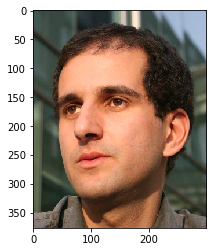Below is the default structure of the element for erosion, which a 1 cross

```np.array([
[0, 1, 0],
[1, 1, 1],
[0, 1, 0]],
bool)
```

Below is the implementation

 `# importing required libraries ` `import` `mahotas ` `import` `mahotas.demos ` `from` `pylab ``import` `gray, imshow, show ` `import` `numpy as np ` ` `  `# loading iamge ` `luispedro ``=` `mahotas.demos.load(``'luispedro'``) ` ` `  `# filtering image ` `luispedro ``=` `luispedro.``max``(``2``) ` ` `  `# otsu method ` `T_otsu ``=` `mahotas.otsu(luispedro) ` `  `  ` `  `# image values should be greater than otsu value ` `img ``=` `luispedro > T_otsu ` ` `  `print``(``"Image threshold using Otsu Mehtod"``) ` ` `  `# showing image ` `imshow(img) ` `show() ` ` `  `# erode strcture ` `es ``=` `np.array([ ` `        ``[``1``, ``1``, ``1``], ` `        ``[``1``, ``1``, ``1``], ` `        ``[``1``, ``1``, ``1``]], ``bool``) ` ` `  ` `  `# eroding image using element structure ` `new_img ``=` `mahotas.morph.erode(img, es) ` ` `  `# showing dilated image ` `print``(``"Eroded Image"``) ` `imshow(new_img) ` `show() `

Output :

`Image threshold using Otsu Mehtod`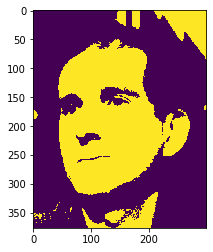`Eroded Image`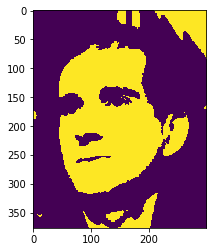Another example

 `# importing required libraries ` `import` `mahotas ` `import` `numpy as np ` `import` `matplotlib.pyplot as plt ` `import` `os ` `  `  `# loading iamge ` `img ``=` `mahotas.imread(``'dog_image.png'``) ` `       `  `# setting filter to the image ` `img ``=` `img[:, :, ``0``] ` ` `  `# otsu method ` `T_otsu ``=` `mahotas.otsu(img) ` `  `  ` `  `# image values should be greater than otsu value ` `img ``=` `img > T_otsu ` ` `  `print``(``"Image threshold using Otsu Mehtod"``) ` ` `  `# showing image ` `imshow(img) ` `show() ` ` `  `# erode strcture ` `es ``=` `np.array([ ` `        ``[``0``, ``0``, ``0``], ` `        ``[``0``, ``1``, ``0``], ` `        ``[``0``, ``0``, ``0``]], ``bool``) ` ` `  ` `  `# eroding image using element structure ` `new_img ``=` `mahotas.morph.erode(img, es) ` ` `  `# showing dilated image ` `print``(``"Eroded Image"``) ` `imshow(new_img) ` `show() `

Output :

`Image threshold using Otsu Mehtod`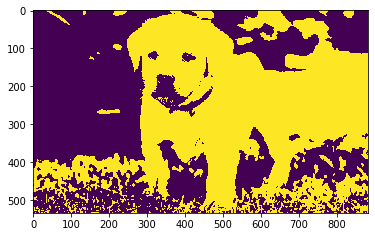`Eroded Image`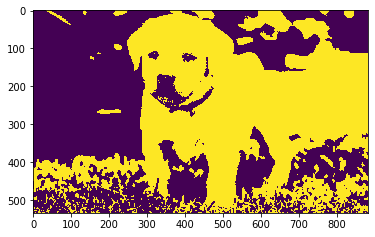Attention geek! Strengthen your foundations with the Python Programming Foundation Course and learn the basics.

To begin with, your interview preparations Enhance your Data Structures concepts with the Python DS Course.

My Personal Notes arrow_drop_upCheck out this Author's contributed articles.

If you like GeeksforGeeks and would like to contribute, you can also write an article using contribute.geeksforgeeks.org or mail your article to contribute@geeksforgeeks.org. See your article appearing on the GeeksforGeeks main page and help other Geeks.

Please Improve this article if you find anything incorrect by clicking on the "Improve Article" button below.

Article Tags :

Be the First to upvote.

Please write to us at contribute@geeksforgeeks.org to report any issue with the above content.